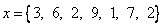## Harmonic Mean

Harmonic mean is the reciprocal of arithmetic mean of reciprocal.

Formula:Properties:

Example:Example applications of Harmonic Means:

Use the interactive program below to compute Harmonic mean of a list of numbers separated by comma. You may change with your own input values.

Input list of positive numbers separated by comma, then press the button "Get Harmonic Mean". The program will compute directly when you change the input data.

Preferable reference for this tutorial is

Teknomo, Kardi (2015) Mean and Average. https:\\people.revoledu.com\kardi\ tutorial\BasicMath\Average\